### MIN Function – Examples in Excel, VBA, Google Sheets

This tutorial demonstrates how to use the Excel MIN Function in Excel to calculate the smallest number.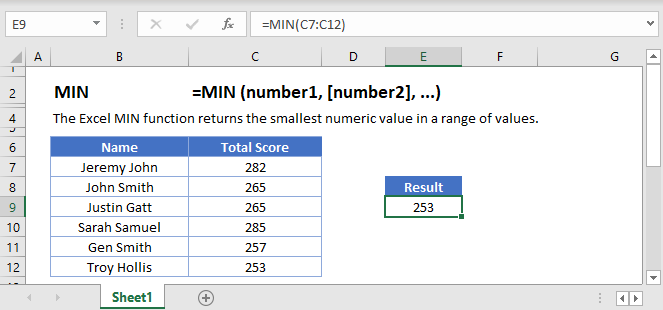## MIN Function Overview

The MIN Function Calculates the smallest number.

To use the MIN Excel Worksheet Function, select a cell and type: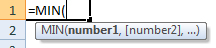(Notice how the formula inputs appear)

## MIN function Syntax and inputs:

`=MIN(number1,number2)`

array – An array of numbers.

AutoMacro - VBA Code Generator

## How To Use The MIN Function

The MIN Function returns the smallest value from a range of values. It is the exact opposite of MAX Function.

Here let’s use the MIN Function to find the lowest textbook price.

`=MIN(B2:B9)`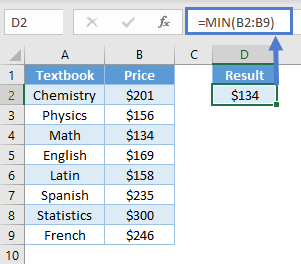### Empty Cells or Cells With Text

The MIN Function ignores cells that are empty or that contain non-numeric values.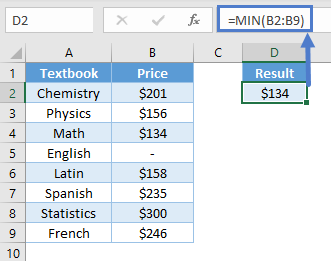## MIN Date

Since Excel stores date as serial numbers, we can use the MIN Function to find the minimum date.

`=MIN(B2:B8)`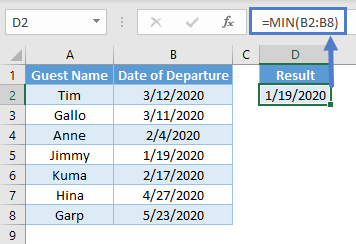## VLOOKUP & MIN

In our above example, we used the MIN Function to find out the lowest textbook price. We can use the VLOOKUP Function to find out the cheapest textbook by using the following formula.

`=VLOOKUP(MIN(A2:A9), A2:B9, 2, 0)`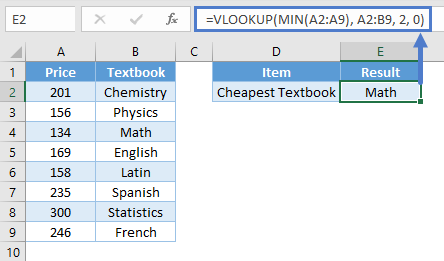Notice how the MIN Function calculates the lowest textbook price and returns that value to the VLOOKUP Function. Afterwards, the VLOOKUP Function returns the textbook associated with the lowest price by searching the second column (as indicated by “2” in our formula) of the table.

Note: For the VLOOKUP Function to work, the textbook prices must be placed in the first column.

## MINIFS Function

MINIFS Function returns the minimum value from a set of values by applying one or more conditions. Let’s say we want to find the lowest textbook price that has been sold out.

`=MINIFS(C2:C9, B2:B9, "Sold")`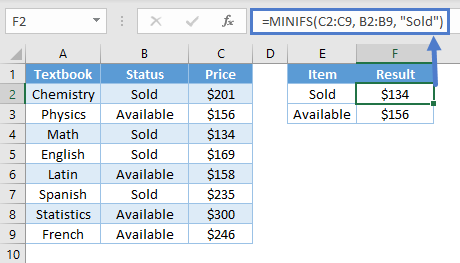Notice how the formula looks for the “Sold” status from B2:B9 and creates a subset of textbook price. The minimum value is then calculated from only the relevant set of data – the sold textbooks.

To learn more about how the MINIFS Function works, read our tutorial on MAXIFS and MINIFS.

Note: MINIFS Function is only available in Excel 2019 or Excel 365. If you have an older Excel version, you can use Array Function to make your own MIN IF.

## MIN IF

If you are using older Excel versions, you can combine IF Function with MIN Function using an Array Function. Taking the same example as above, use the formula:

`=MIN(IF(B2:B9 = "Sold", C2:C9))`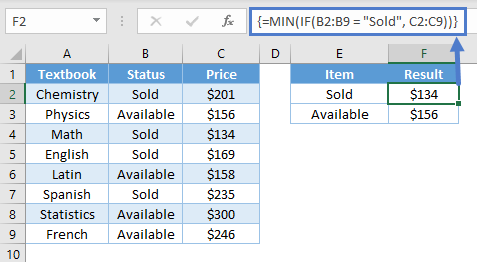Note: When building array functions, you must press CTRL + SHIFT + ENTER instead of just ENTER after creating your formula.

You’ll notice how the curly brackets appear. You can not just manually type in the curly brackets; you must use CTRL + SHIFT + ENTER.

The IF Function helps narrow down the range of data and MIN Function calculates the minimum value from that subset. In this way, the price for the cheapest text that has been sold can be calculated.

Note: The above formula uses absolute references (the \$ signs) to lock cell references when copying formulas. If you aren’t familiar with this, please read our Excel References Guide

## MIN function in Google Sheets

The MIN function works exactly the same in Google Sheets as in Excel.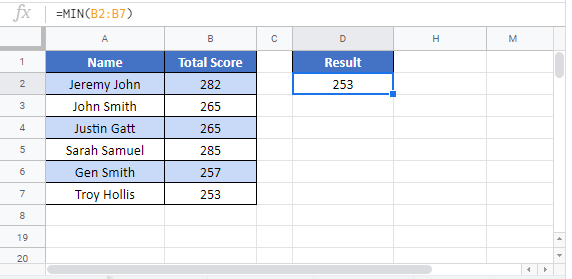## Additional Notes

The MIN Function returns the smallest number in a series. Logical values (TRUE and FALSE), and numbers stored as text are not counted. To count logical values and numbers stored as text use MINA instead.

## MIN Examples in VBA

You can also use the MIN function in VBA. Type:
`application.worksheetfunction.min(number1,number2)`
For the function arguments (array, etc.), you can either enter them directly into the function, or define variables to use instead.

Assuming that we have the following range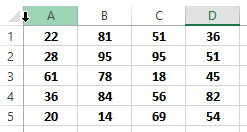we can get the minimum number in th range A1:D5 with the following VBA statement

`WorksheetFunction.Min(Range("A1:D5"))`

Which would return 14, as this is the smallest number in that range

The MIN function can also accept a table as a parameter, so the following statement is valid as well, provided that there is a table named “Table1” in our worksheet

`WorksheetFunction.Min(Range("Table1"))`

We can also use the MIN fuction by directly entering numbers as parameters, like the following example

`WorksheetFunction.Min(1, 2, 3, 4, 5, 6, 7, 8, 9)`

which will return 1 as a  result

Return to the List of all Functions in Excel

## Excel Practice Worksheet

Practice Excel functions and formulas with our 100% free practice worksheets!

• Automatically Graded Exercises
• Learn Excel, Inside Excel!